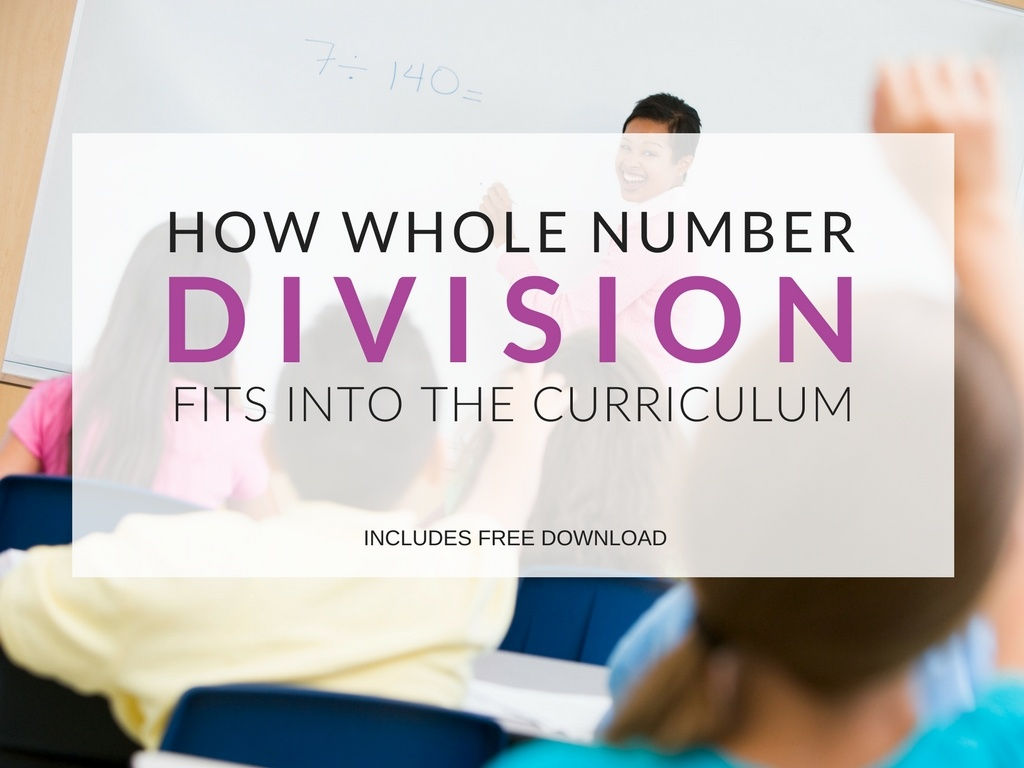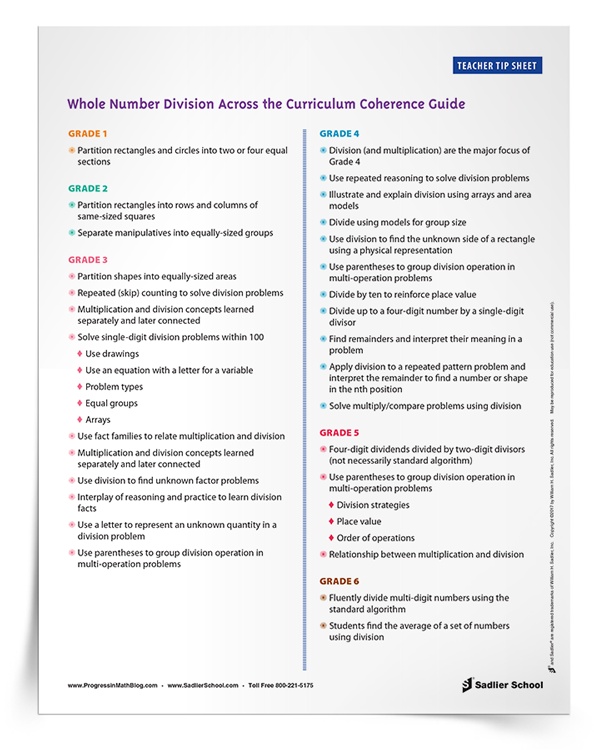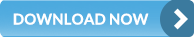Shop Now Login/Register View Quote View Cart
1.800.221.5175
Mathematics
Core Math
Supplemental
Let's Target Math Problem Solving Grades 1–8 View Details | Buy Now
Let's Target Real-World Math Word Problems Grades 1–8 View Details | Buy Now
Critical Thinking for Active Math Minds Grades 3–6 View Details | Buy Now
Vocabulary
Vocabulary Workshop Achieve Interactive Edition Grades 6–12+ View Details | Buy Now
Let's Target Vocabulary and Usage Grades 1–8 View Details | Buy Now
English Language Arts
Grammar & Writing

A K–8 resource to support deep comprehension of math skills and conceptsMarch 21, 2017 other-professional-development

Long division is one of the most difficult tasks for late elementary and middle school students to learn. While many students master it quickly, for others it can be a painful process.

This is the second article in this series about how mathematical topics are related across the grade levels. It provides insight into what students learn at each grade level, how the topic of division develops over years, and how you can focus on the specific tasks at hand for your grade.If you are working as a math coach or curriculum coordinator, or are mentoring a teacher at a different grade level, this post’s associated download will be useful in one-on-one, grade level, cross-grade, or departmental meetings.

It can serve as a starting point for discussions that are aimed at attaining coherency across and between grade levels.

In addition, knowing the trajectory of curriculum, will allow teachers to identify students who are falling behind and design interventions to help them.

WHOLE NUMBER DIVISION ACROSS THE CURRICULUM

Although students are expected to use the standard algorithm fluently by the end of Grade 6, the foundation for division begins in Grade 1, and the conceptual development of the concept of division begins in third grade and is a major focus of Grade 4.

In Grade 1, students are dividing figures into halves and fourths. The partitioning of shapes in Grades 1 and 2 should focus on the idea that the shapes have equally-sized partitions.

In Grade 2, students begin to create rectangular arrays and to separate manipulatives into equally-sized groups. The basis of division is conveyed as splitting shapes or a group of items into equally-sized parts.

A key concept that transcends math in many ways is the idea that division and multiplication are inverse operations. It is important that the concepts of multiplication and division are taught separately first, but by the end of Grade 3, students should be able to articulate this relationship even simply by being able to write fact families consisting of related two multiplication and two division sentences.

In Grade 3, Students should learn a variety of strategies in order to accomplish division. These include separating objects into equally-sized groups, skip counting by the divisor to find the dividend, or creating arrays with objects or by drawing. Teachers should employ a balance of reasoning and practice in order for students to learn their division facts. Letters serve as variables that are used as unknowns in order to solve unknown factors by division.

In Grade 4, multiplication and division are the major mathematical focus. The outcome of Grade 4 is that students can divide up to a four-digit number by a single-digit number. This brings in the idea of remainders. Students also find the nth number, figure, or letter in a pattern. For instance, the 100th member in a three-member pattern can be found by dividing 100 by 3 and interpreting the remainder. The relationship of multiplication and division to area and area models is one way that students solidify the relationship between the two operations.

In Grade 5, students are beginning to formalize the process of dividing, though they still use drawing, arrays, and non-standard procedures to find quotients. They divide by two-digit numbers. The expectation for using the standard algorithm, while an admirable goal for Grade 5 students, is not an expectation until Grade 6. Students begin to learn to divide decimals in addition to whole numbers.

By Grade 6, students learn the standard algorithm for dividing whole numbers (and decimals). An application of division is finding the average (mean) of a set of numbers.

You can download the Whole Number Division Coherence Guide to see the details of what will be learned at each grade level. Print it out or share it today to spark conversation among your staff and colleagues.IN SUMMARY

Knowing the trajectory of division helps teachers maximize their impact by focusing on exactly what needs to be taught! Having a command of how the backbone of mathematics curriculum is constructed will help math coaches or curriculum coordinators support teachers.

Help teachers under your stewardship understand the trajectory of division across grade levels so they will know exactly what to teach!